# Sum by OFFSET groups in Rows and Columns

In this article, we will learn how to Calculate Sum by groups in Rows and Columns in Excel.

For Instance, you have a large list of data and you need to find the SUM of the numbers having a set of n values .

For this article we will be needing the use the following functions:

1. SUM Function
2. OFFSET function

SUM function is a built - in mathematical function which returns the SUM of numbers given argument to the function
Syntax:

= SUM ( number1 , [number2], ...)

The OFFSET function returns a cell or specified number of range of cells traversed through a cell reference.
Syntax:

= OFFSET ( reference , rows , columns , [height] , [width] )

Reference : cell reference to start from
Rows : number of rows traversed from the cell reference
Columns: number of columns traversed from the cell reference.
[height] : height or number of cells in the column to pick.
[width] : width or number of cells in the row to pick.

First 3 arguments of the function is the path to the required cell.
4th & 5th argument of the function indicates the number of cells to return.
Now we will make a formula out of these functions. Here we will given the data and we needed to find the SUM having a specified number of cells.
Use the formula:

= SUM ( OFFSET ( reference , ro , co , he , wi ) )

Explanation:

• OFFSET function starts from the reference and traverse ro number of rows and co number of columns from the cell reference.
• Now the function starts picking groups of numbers having he number of values in the column and we number of values in the row.
• The OFFSET function now returns the array of numbers to the SUM function.
• SUM function adds up all the numbers and returns the SUM.

Let's test this formula via running it on an example

Here we have a data from A1 : D16. we need to find the SUM of Quantity & Total Price in a group of 5 with the given reference from the A1 cell.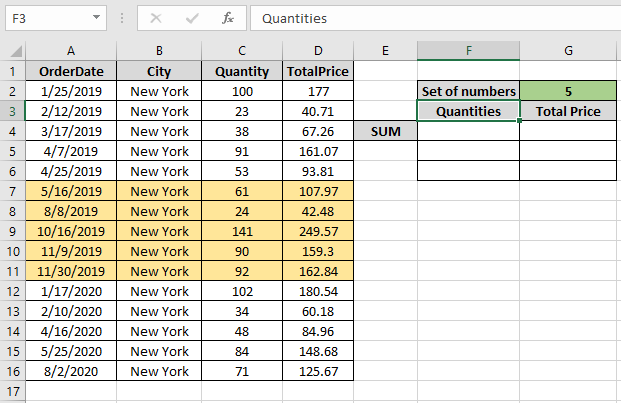First we need to find the SUM of first 5 quantity which are values { 100 , 23 , 38 , 91 , 53 }

Use the Formula:

= SUM ( OFFSET ( A1 , 1 , 2 , 5 , 1 ) )

Explanation:

• OFFSET function starts from the A1 cell and reaches the C2 cell traversing the 1 row and 2 columns.
• We need first 5 cells from C2 to C6 cell. So the height 5 and width will return us the numbers as shown below.

= SUM ( { 100 ; 23 ; 38 ; 91 ; 53 } )

• Now the SUM function returns the SUM of the values.

Here the A1 is given as cell reference & rest of the arguments are provided directly to the function.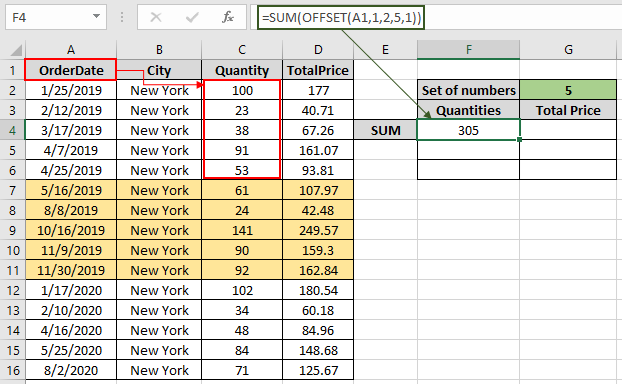As you can see in the above snapshot the SUM of first five quantity comes out to be 305 .
Now we will get the SUM for all the other groups of 5 by changing the Row argument of the function

Use the Formula:

= SUM ( OFFSET ( A1 , 6 , 2 , 5 , 1 ) )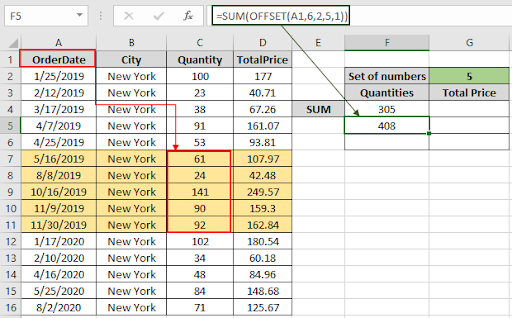As you can see the SUM of next five in the snapshot above. For the next five change the row argument to 11.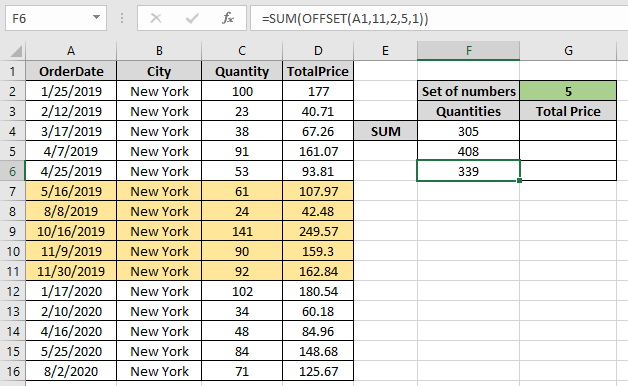Get the SUM of TotalPrice for the same by changing the Column argument to the function.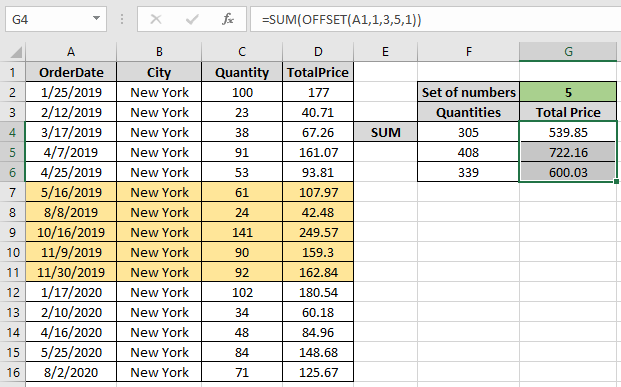As you can see from the above formula that you can get the SUM values by groups using the OFFSET function.

Notes: The function returns #VALUE! error if the argument to the function is non-numeric.

Hope this article about how to Sum by OFFSET groups in Rows and Columns in Excel is explanatory. Find more articles on SUMPRODUCT functions here. Please share your query below in the comment box. We will assist you.

Related Articles

How to use the OFFSET function in excel

How to use the SUM function in excel

How to use the ISFORMULA function in Excel

How to Remove Text in Excel Starting From a Position

How to use the ROWS function in Excel

Validation of text entries

Create drop down list in excel with colour

Remove leading and trailing spaces from text in Excel

Popular Articles

Edit a dropdown list

Absolute reference in Excel

If with conditional formatting

If with wildcards

Vlookup by date

Join first and last name in excel

Count cells which match either A or B

Convert Inches To Feet and Inches in Excel 2016

50 Excel Shortcut to Increase Your Productivity

1.Sir i have a excel sheet plz tell me about sum offset function
A1 5
A2 10
A3 15
A4 20
A5 25
A6 30
If i enter value in B1 2 then get total of A1 and a2 in C1
If i enter value in B1 3 then get total of A1 A2 and A3 in C1
If i enter value in B1 4 then get total of A1 A2 A3 and A4 in C1
If i enter value in B1 5 then get total of A1A2A3A4 and A5 in C1
Plz tell m formula

•Hi vikas,
Thanks for reaching out.

Write this formula in Cell C1,
=SUM(OFFSET(A1,0,0,B1))

This will sum up the given number of cells in B1. The sum will start from A1 to number of cells below.

Terms and Conditions of use

The applications/code on this site are distributed as is and without warranties or liability. In no event shall the owner of the copyrights, or the authors of the applications/code be liable for any loss of profit, any problems or any damage resulting from the use or evaluation of the applications/code.